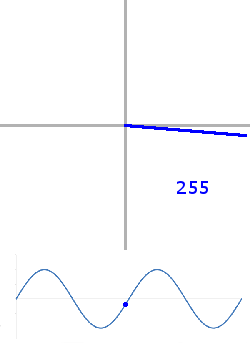You may also be interested in our article regarding fixed-point math!

# Intro

Division and modulo operations should be avoided on 8- and 16-bit hardware.  We would like to present you with a few techniques that can help you avoid the cycle-heavy division operations by replacing your modulo operators with bit masks and your division operations with bit shifts.  I have focused on 'C' for this article, but they are just as valid in all programming languages.

# Powers of 2

You will see a recurring theme in this article, powers of 2 make your life easier.  This is for the same reason that powers of 10 make your life easier in the metric system.  Math just tends to work out easily.  Whenever possible, make your multiplication, division, length, whatever a power of 2 and you will thank yourself later.

# Look-up Tables

The processors that we are accustomed to working with on this publication are lumbering, slow devices that can often take microseconds to perform the simplest operations (that is a long time, btw).  As a result, designers often place look-up tables (LuTs) within the processor to replace potentially time-consuming maths.When choosing the length of your table, you should generally choose a length that is a power of 2, especially for periodic waveforms.  Why? Lets take the example of a repeating waveform, 'sine'.  As you know, a sine wave repeats every rotation.  Once it gets to its maximum value, it simply repeats again.  We can take advantage of this using a modulo technique that can be completed very quickly on most processors without doing the first divide, but only if the length of the table is a power of 2.  Keep reading... we come back to this in a moment.

# To Roll or Not to Roll

The most common experience for most people involving a fixed number of digits rolling over is the odometer in a typical car.  If you can find an old Bronco 2 from the early eighties, you will see that they only had 5 digits to work with.  When the old car got to '99999' and then went one more mile, the miles were suddenly '00000' again.  This is exactly the modulo operation.  In this case:

 `1` ```odometer = miles % 100000; ```

We have similarly easy operations on our simple processors, but we try to implement them in the easiest and most straightforward way.  Just as the odometer in the base 10 example was very easy to modulo with a power of 10, it is just as easy to modulo a binary number with a power of 2;

Going back to our sine LuT, we can traverse through our table by adding 1 and looking up the next value.

 ```1 2 3``` ```output = sineTable[sineIndex]; sineIndex++; ```

There will be a point when 'sineIndex' will step beyond the bounds of the lookup table.  This is where our modulo operation can help.  By using the modulo operator, we cause the lower order bits to repeat continually, just as the above animation illustrates.  Assuming a 256-element lookup table:

 ```1 2 3``` ```output = sineTable[sineIndex % 256]; sineIndex++; ```

There are several compilers that will pick this up as a modulo operator on a power of two and insert the appropriate optimization.  It is this author's opinion that the programmer should be able to recognize the optimization himself and state his desire more clearly as all compilers do not recognize the optimization:

 ```1 2 3``` ```output = sineTable [sineIndex & 0x00ff]; sineIndex++; ```

This set of statements has the exact same effect as the previous set of statements and completes the argument on why your LuT length should be a power of two.  If your sine-table length would have been anything other than a power of two length, then this optimization would not have been possible.

Alternatively, for very special cases which happen to line up with compiler defined lengths, you can forego the mask and the rollover will occur automatically for the same reason that it occurred on your odometer:

 ```1 2 3``` ```uint8_t sineIndex;   // this is only an 8-bit number output = sineTable[sineIndex++];   // no mask required ```

# Feeling Shifty

Division by any power of two can take place using the simple shift operation '>>':

 `1` ```output = 12 >> 1; ```

is the same as:

 `1` ```output = 12 / 2; ```

Most of you know this, but we can take it further.  This division is the same as multiplication by 1/2:

 `1` ```output = 12 * 1/2; ```

What if you wanted to multiply by 3/4?  As long as your divisor is a power of two or can be broken down into a sequence of powers of two, you can use the shift operation to your advantage.  Below, each expression is exactly equivalent to the others:

 ```1 2 3 4 5``` ```output = 12 * 3/4; output = (12 * 1/2) + (12 * 1/4); output = (12 >> 1) + (12 >> 2); ```

On some compilers, the upper most expression would institute a multiplication then a division operation, which will cost you tens of cycles (hundreds on some).  The bottom expression will always end up being a couple of bit shifts and an add.

# Summary

To perform modulo operations, use bit masks or use the maximum value allowed for that variable type.  To perform divisions, use bit shifts as much as possible.  Remember to use powers of 2 throughout your code.  As always, have fun!

© by Jason R. Jones 2016
My thanks to the Pelican and Python Communities.